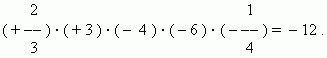# Operations with negative and positive numbers

Absolute value (modulus) of a number. Addition.
Subtraction. Multiplication. Division.
Rules of signs at multiplication and division.

Absolute value (modulus): for a negative number this is a positive number, received by changing the sign " – " by " + "; for a positive number and zero this is the number itself. The designation of an absolute value (modulus) of a number is the two straight brackets insideof which the number is written.

E x a m p l e s :

| – 5 | = 5, | 7 | = 7, | 0 | = 0.

 Addition: 1)  at addition of two numbers of the same sign their absolute values are added and before the sum their common sign is written. E x a m p l e s : ( + 6 ) + ( + 5 ) =   11 ; ( – 6 ) + ( – 5 ) = – 11 ; 2)  at addition of two numbers with different signs their absolute values are subtracted(the smaller from the greater) and a sign of a number, having a greater absolute value is chosen. E x a m p l e s : ( – 6 ) + ( + 9 ) =    3 ; ( – 6 ) + ( + 3 ) = – 3 .

Subtraction: it is possible to change subtraction of  two numbers by addition, thereat a minuend saves its sign, and a subtrahend is taken with the back sign.

E x a m p l e s :

( + 8 ) – ( + 5 ) = ( + 8 ) + ( – 5 ) =      3;
( + 8 ) – ( – 5 ) = ( + 8 ) + ( + 5 ) =   13;
( – 8 ) – ( – 5 ) = ( – 8 ) + ( + 5 ) = –  3;
( – 8 ) – ( + 5 ) = ( – 8 ) + ( – 5 ) = – 13.

Multiplication: at multiplication of two numbers their absolute values are multiplied, and a product has the sign " + ", if signs of factors are the same, and  " – ", if the signs are different. The next scheme ( a rule of signs at multiplication ) is useful:

+   ·   +   =   +
+   ·   –   =   –
–   ·   +   =   –
–   ·   –   =   +

At multiplication of some factors (two and more ) a product has the sign " + ", if a number of negative factors is even, and the sign " – ",  if this number is odd.

E x a m p l e :Division: at division of  two numbers the first absolutevalue is divided by the second and a quotient has the sign " + ", if signs of dividend and divisor are the same, and " – ", if they are different. The same rule of signs as at multiplication acts:

+   :   +   =   +
+   :   –   =   –
–   :   +   =   –
–   :   –   =   +

E x a m p l e :

( – 12 ) : ( + 4 ) = – 3 .